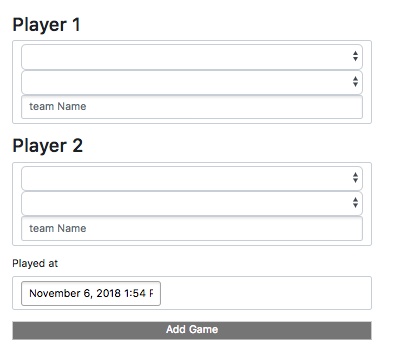Build an ELO platform with Re-Frame

Created: 2018-11-28 Wed 14:51

Who Am I

• senior software developer at Funding Circle
• API & backends for a long time
• saw the light with Clojure & Clojurescript

PlayEloElo rating system

• method for calculating the relative skill levels of players
• can be applied to any zero sum game (chess / tennis / table tennis…)
• new rankings only depends on current rankings and result
• points won or lost depend on the ranking difference between two players
• average of all the points is constant

Elo example

A: 1500, B: 1500

$$E\_A = \frac{1}{1 + 10 ^ \frac{RB - RA}{400}} = \frac{1}{1 + 10 ^ \frac{0}{400}}$$ $$E\_A = \frac{1}{2} = 0.5$$ $$E\_B = \frac{1}{2} = 0.5$$

A vs B (3-0):

$$R\_A = 1500 + (K * (1 - E\_A)) = 1500 + (32 * (1 - 0.5)) = 1516$$ $$R\_B = 1500 + (K * (1 - E\_B)) = 1500 + (32 * (0 - 0.5)) = 1484$$

A = 1516, B = 1484

Elo implementation (1)

$$E\_A = \frac{1}{1 + 10 ^ \frac{RB - RA}{400}}$$

$$R\_A = R\_A + (K * (1 - E\_A))$$

(defn expected
[diff]
(/ 1.0 (inc (Math/pow 10 (/ diff 400)))))

(defn new-rating
[old expected score]
(+ old (* k (- score expected))))

Elo implementation (2)

(defn new-rankings
[rankings [p1 p2 score]]

(let [ra (get rankings p1)
rb (get rankings p2)]

(assoc rankings
p1 (new-rating ra
(expected (- rb ra))
score)

p2 (new-rating rb
(expected (- ra rb))
(invert-score score)))))

;; P1 wins against same level opponent:
(new-rankings {:p1 1500 :p2 1500} [:p1 :p2 0])
;; => {:p1 1484.0, :p2 1516.0}
;; P1 wins against much stronger opponent:
(new-rankings {:p1 1300 :p2 1700} [:p1 :p2 1])
;; => {:p1 1329.090909090909, :p2 1670.909090909091}

Re-frame

re-frame is a pattern for writing SPAs in ClojureScript, using Reagent.

• React
• Reagent
• Re-Frame

Reagent Syntax

JSX

function getGreeting(user) {
if (user) {
return <h1>Hello, {formatName(user)}!</h1>;
}
return <h1>Hello, Stranger.</h1>;
}

REAGENT

(defn get-greeting
[user]
(if user
[:h1 [str "Hello" [format-name user]]]
[:h1 "Hello, Stranger"]))

Reagent rendering

const element = <h1>Hello, world</h1>;
ReactDOM.render(element, document.getElementById('root'));
(def element [:h1 "Hello, world"])

(reagent/render-component element
(.-getElementbyid js/document "root"))

Re-frame in action

Re-frame primitives

• subscriptions: reg-sub
• event handler: reg-event-db
• effect handler: reg-event-fx

FormDB

MODEL

(def default-game
{:p1 ""
:p2 ""
:p1_points ""
:p2_points ""
:p1_using ""
:p2_using ""
:played_at (js/moment)})

Subscription

CONTROLLER

(rf/reg-sub ::game
(fn [db _]
[::game db]))

(rf/reg-event-db ::p1_using
(fn [db [_ val]]
(assoc-in db [::game :p1_using] val)))

VIEW

(let [game @(rf/subscribe [::handlers/game])]
[:input.form-control
{:type "text"
:placeholder "Name"
:value (:p1_using @game)
:on-change (utils/set-val ::handlers/p1_using)}])

API Call

(rf/reg-event-db
::on-success
(fn [db [_ games]]
(assoc db ::games games)))

(rf/reg-event-fx